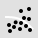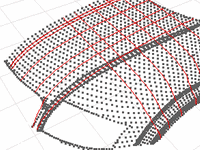# PointCloudSectionCurve

Curve from Objects>

Section Through Points

The PointCloudSection command creates a planar curve by intersecting a plane with points or pointcloud objects.#### Steps

1. Select point or point cloud objects, and press Enter.
2. Pick the start of the section plane.
3. Pick the end of the section plane, and press Enter.
Section curves are created by intersecting the points with the section plane, which is perpendicular to the construction plane.

Point Cloud Section Options

#### Point sampling options

##### Maximum distance to plane

The thickness of the "slab" around the plane from which sample points are taken. Those sample points are projected to the section plane and a polyline is found that connects them. This distance depends on the size of the point cloud and the spacing of the points.

##### Minimum distance between points

A threshold for the minimum spacing between adjacent sample points. If there are points closer than that, some are not used.

#### Curve creation options

##### Create smooth curves

Creates a smooth curve. You can create both a curve and a polyline.

##### Create polylines

Creates a polyline. You can create both a curve and a polyline.

##### Create open/closed curves

Creates a open or closed curves.

##### Fitting tolerance

The tolerance used to fit the curve through the polyline.

# PointCloudContourCurve

Curve from Objects>

Contour Through Points

The PointCloudContour command creates a series of planar curves by intersecting planes with points or pointcloud objects.#### Steps

1. Select point or point cloud objects, and press Enter.
2. Pick the contour plane base point.
3. Pick the direction perpendicular to the contour lines, and press Enter.
Contour curves are created by intersecting the points with the contour planes, which are perpendicular to the construction plane.
Command-line option

Range

Specifies limits for the contours.

#### Steps

1. Select objects for contour creation.
2. Pick the start for the contour curves and points.
3. Pick the end of the contour range perpendicular to the contour planes.
The range line determines the direction for the contours curves.
The contour planes will be perpendicular to this direction, generated from the base point to the end point.
4. Specify the distance between contours and press Enter.
Contour curves are created where the contour planes intersect the objects.

Point Cloud Contour Options

#### Point sampling options

##### Maximum distance to plane

The thickness of the "slab" around the plane from which sample points are taken. Those sample points are projected to the contour plane and a polyline is found that connects them. This distance depends on the size of the point cloud and the spacing of the points.

##### Minimum distance between points

A threshold for the minimum spacing between adjacent sample points. If there are points closer than that, some are not used.

#### Curve creation options

##### Create smooth curves

Creates smooth curves. You can create both curves and polylines.

##### Create polylines

Creates polylines. You can create both curves and polylines.

##### Create open/closed curves

Creates a open or closed curves.

##### Fitting tolerance

The tolerance used to fit the curve through the polyline.

##### Contour distance

The distance between the contour lines.